# Introduction

In this article I’ll present a beginner-oriented framework implementing neural networks in C++. The main goal of this code is to understand the root of neural networks for beginners, it also allows multiple modifications which are usually hard to implement in classical high-level framework (Pytorch, Tensorflow, Keras etc..).

So this code can also be used for some research because you can also do things you couldn’t do in other frameworks.

1. Features of the framework
2. Neural networks from scratch
3. Framework design
4. Exotic backpropagation-free optimizer: Shaking Tree optimizer
5. What I learned doing this
6. Github repository

Let’s go!

# 1. Features of the framework

• Graph implementation of neural network (no matrices)
• Neural network, neurons and edges as explicit objects
• Only multilayer perceptrons with Linear/ReLU/Sigmoid activations
• No parallel computations, no optimizations, no convolutional layers
• Exotic backpropagation-free optimizer

# 2. Neural networks from scratch

Neural networks can be interpreted in two ways. The “graph” way and the “matrix” way. When you begin with neural networks, people usually teach you the “graph” way:

That’s how neural networks are usually represented, vertices(neurons) + oriented edges(dendrites), a directed graph. But in practice they’re used with matrices because computations are much faster on GPUs that way.

For complete beginners, you can try playing with neural network on this webpage. The easy version of how a neural network does the forward pass is as follows:

(0) create a neural network following the above architecture

(1) put values in the input neurons (exp: 3 neurons with value 1, 5 and 3)

(2) trigger the input layer. The values are multiplied with a weight linking each input neuron to each neuron of the 2nd layer (3neurons*4neurons=12scalars/weights/edges). Then sum the 3 inputs*weights for each of the 4 neurons of the 2nd layer. (exp: 1*a1+5*b1+3*c1 for 1st neuron of 2nd layer, then 1*a2+5*b2+3*c2 etc.)

(3) trigger the 2nd layer and do (2) between the 3rd layer and the 2nd layer (the same way it was done between the 2nd and the 1st layer)

(4) read the two values accumulated by the 3rd/output layer

The forward pass is easy. The same computations can be done with matrices. What’s harder to understand and important to re-implement is the backward pass, the method used to learn the right weights. The inputs [1, 5, 3] and outputs of each layer depend on the data we’re processing. The weights [a1, b1, c1, a2..] are here to process all inputs correctly. We’ll focus on finding the good weights.

In this repo, neural networks are implemented in a graph way. Some Github repositories of that kind exist because people - including me - think it’s a great way to be sure you understood how neural networks work, and it’s also a great way to play with neural networks.

This repo is a beginning of neural network framework in C++ because it’s more elaborated than “just the neural-network part” in one file. It’s not even 0.01% of what libs like dlib are, and it’s not meant to be a framework that would serve anything but playing around and understanding neural networks.

# 3. Framework Design

• Neural: Contains classes related to the neural network
• Dataset: Contains one simple class for reading a dataset
• Optimizer: Contains a generic optimizer class, a classical backpropagation optimizer and an exotic optimizer
• Misc: Contains activation functions and their derivatives

2. Neural Classes

• NeuralNetwork.h/.cpp: the main class containing a model. A NeuralNetwork is composed of multiple layers
• Layer.h/.cpp: A Layer is composed of multiple neurons. It has a type (INPUT/STANDARD/OUTPUT) because it doesn’t work the same way depending on its type and it has an activation function
• Neuron.h/.cpp: A Neuron has a parent layer. A neuron accumulates the output of the edges connected to it (_accumulated), it outputs that input to its edges after processing it with its _activation_function (which you can change on a per-neuron basis). A neuron knows its _next and _previous edges.
• Edge.h/.cpp: An Edge knows its next neuron (_n), the neuron its coming from (_nb), it has a weight (_w), it can memorize how much its weight was shift (_last_shift) last time it was, and it has a backpropagation memory so that it can retain a part of the chain rule

3. Dataset / Optimizer

• Dataset.h/.cpp: It gives the data. One input/output is just a vector (again, no matrices, no images). So the list of all inputs is a vector of vector. The Dataset class is also responsible for doing the train/test split.
• Optimizer.h/.cpp: Abstract class that links the network and the dataset together. Child classes must implement a “minimize” method that is called during the optimization process to minimize the loss.
• Backpropagation.h/.cpp: Implementation of the backpropagation optimizer (though the backpropagation computations are in the neuron class for simplification). This class is mostly there to call these methods in the neuron class and to apply the new computed weights. It uses a batch size, the learning rate is a global variable because it’s common to all implemented optimizers and it’s easier to access it from anywhere.
• ShakingTree.h/.cpp: Exotic optimizer that’ll be detailed later.

## Computation example

The algorithm I provide was also made to exactly reproduce the “famous” article from Matt Mazur on his website: https://mattmazur.com/2015/03/17/a-step-by-step-backpropagation-example/

Just use the “mattmazur_step_by_step” branch on git, this branch serves only that purpose and contains older code than the code on “master”, but the backpropagation part works the same way.

You can add logs wherever you want to understand the computations following the blog post from mattmazur. Mostly you can uncomment the cout line in the “getBackpropagationShifts” method in neuron.cpp.

As you can see, the results are exactly the same he got.

## Building a Neural Network

It’s pretty much straightforward:

Instanciate the class. Add an input layer, specify the number of neurons (size). Then add hidden layers (standard), specify the number of neurons (size=5 neurons) and an activation function (sigmoid). Finally, add an output layer, its size (1 output value) and an activation function (sigmoid).

Yeah, C++ can have a quite great readability now.

The “autogenerate” adds the edges (connectComplete) so that the layers are fullyconnected, and it also randomizes all the weights:

## Predicting a value

That’s also an easy one:

The inputs are set up in the _accumulated variable of the input neurons, then trigger() calls each layer to fire successively, it computes the output of that layer and put it in the input of the neurons in the next layer. Finally the output() just returns the activation_function(accumulated) for each neuron of the last/output layer.

## Backpropagation

The chain rule is implemented in the getBackpropagationShifts() methods in neuron.cpp.

___ About backpropagation. Going into details wouldn’t serve much as you mostly only need to read the guide from mattmazur to understand what’s being done in here mathematically.

Put simply, backpropagation is about computing how to alter the weights such that the output of the network corresponds to the data you’re trying to do a prediction on. If we have high values in the output but the values we’re trying to predict are close to zero, backpropagation will make the weights closer to zero.

How? We have the output we made and the right prediction (the target). We can predict the error between these two (error = target - output). And as everything is only a succession of mathematical operations like y = f(x) and is differentiable, we can compute the partial derivative of the error with respect to the weights (basically Δerr/Δw).

Which means we know how influencing a weight can influence the error, for the current data we’re reading and based on the weights we were using.

(new_weight) will be (old_weight - Δerr/Δw).

___ About the code. You need the target (the values we’re trying to learn) to do the computations for the output layer (the “if” block), then you need to keep in memory a part of the computations to apply the chain rule in all other layers (the “else” block).

dw stands for delta_weight, so for each neuron you’re essentially computing a delta to apply to each of its _previous edges, it’s backpropagation starting from the output layer.

The chain rule puts Δerr/Δw in three operations which gives the three d1, d2 and (d3) the previous output you have in the code (the line in if section with dw[i] = …). Basically dw[i] = Δerr/Δw.

Functions are named so that they should be easy to read and to understand.

That’s basic backpropagation. No momentum, no tricks, nothing but gradients. The learning rate applies when we add the delta_weight to the weight (shiftBackWeights method of Neuron calls shiftWeight of Edge).

# 4. Exotic backpropagation-free optimizer: ShakingTree optimizer

Here I’ll introduce a new kind of exotic optimizer which I named “Shaking Tree optimizer”.

Why “ShakingTree”? Because we’re going to randomly change the weights, like if we were shaking the graph as a tree or if we were randomly shaking some branches so that only the weaker ones are replaced by greater ones.

## Theory

The goal is of course to do better than a backpropagation algorithm. How does backprop work? (1) do the forward pass (2) compute the loss (3) do complex computations to evaluate the gradients (4) loop.

Can you change the weights of a neural network without doing a forward pass to compute the loss? Well at some points you need to evaluate what you’re doing and how it impacts the loss (1)+(2), so the main thing I wanted to remove is the gradients computations (3).

But I still need a way to change the weights. Ok, some people tried genetic algorithms, it works but it’s quite random, and of course the more weights, the harder it gets to find the right values...but that’s also true with vanishing gradients. We know that gradients give a great estimation of how to change the weights of a neural network. The main idea of ShakingTree is to try to approximate the gradients (OR something that would be at least as great as gradients) from random values without explicitly computing the gradients.

So, is it worth it to quickly try random values? Or is it better to slowly compute gradients? Is there a way to be quick and compute “random” values that end up being better than the gradients? That’s what I tried to answer.

I tried two methods, a “basic” one and a “complex” one.

1. Basic method
• Evaluate the score with current weights on a part of the dataset
• Save current weights
• Apply new weights randomly (amplitude, distribution, number of weights etc.. many hyperparameters)
• Re-evaluate the score on (another?) part of the dataset
• If the new score is worst, re-apply old weights

2. Complex method

• Evaluate the score with current weights
• Generate a delta to be applied to all weights (this delta is meant to act like the gradients)
• Apply the delta weight, evaluate it, save the delta score and the delta weight
• Now technically you can evaluate a kind of f(delta_weight) = delta_score (that’s what the gradients aim to be!)
• Each N iterations, average the delta_weight of the weights that reduced the score (delta_score < 0), and apply that delta

That’s all for the theory, now you know the idea, what are the resuls?

## In practice

The dataset contains two 2d-gaussian distribution, so input is size 2 and output is size 1 (<0.5 is first gaussian, ≥ 0.5 is the second one), so that’s far from any real-world use case for deep learning algorithms. I don’t tune hyerparameters much. Meaning I’m pretty sure any of these curves could be higher or lower than the others with tuning but as we’re not in a real-world use case, it doesn’t really matter. The neural network contains five 10-neurons hidden layer with sigmoid activations (again, probably far from real-world use case).

The plots:

Why is it noisy? Because it’s random and it can sometimes quickly get to bad results. The fact that it goes from bad results to good results partially shows how easy the task is (but changing the final output layer parameters’ can also quickly give bad results and changing them back again can give good results again quickly). minimizeBasicLarger2 and minimizeBasic use the “basic” method of ShakingTree with different hyperparameters. Backpropagation is in blue and the complex version of ShakingTree is in red.

The first hyperparameters I chose for minimizeBasicLarger gave much more random results so I changed them once or twice, giving the green curve. I didn’t change the parameters of the other algorithms on that particular task.

Keeping only the best iteration: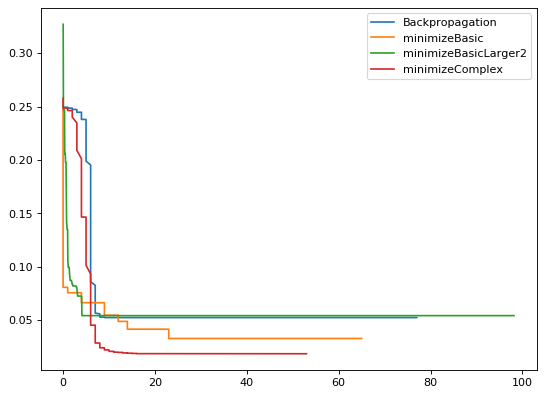Best loss on test set = f(seconds) on a dedicated machine

## Limitations

Just to be clear, be sure that these algorithms do bad outside of their confort zone, which, again, to be honest, would be the confort zone of many other algorithms that don’t scale. For example, the minimizeBasic algorithm changes one weight per iteration, which is ok in a neural network with 500 weights but you sure can’t use that version for a neural network with 5M parameters. And if you randomly change 500.000 weights, you’re less prone to find a great configuration by chance, meaning you have to use smarter metrics.

These ShakingTree algorithms with their worst hyperparameters could probably look like a random search but the added hyperparameters are there to avoid being too close to a random search. These algorithms could probably also be approximated by genetic algorithms, but the same apply, it’s not exactly the same algorithm.

We’re not doing much better than any algorithm that probably performs well on MNIST and actually proving that these algorithms work on these datasets is far from being able to prove that they work on real-life datasets. But maybe with some hyperparameter tuning and with a more tensor-oriented approach it could be applied to convolutional filters on a more standard framework like Pytorch.

This part of the article is mainly a proposition for a new backpropagation-free algorithm. There are many other backprop-free algorithms that could perform better on small datasets with small networks, but which probably are always worst than backprop on larger datasets with larger networks.

# 5. What I learned doing this

• Tensor computations on GPUs and optimizations are probably the only reasons why Deep Learning became that big. I can easily train a model with 15M parameters on my server with GPUs but I can barely train a 20k parameters model on my framework with one thread on one CPU.
• Backpropagation is very efficient because it’s two operations in one. When doing backprop, you’re evaluating a way to change your parameters AND evaluating the score of your parameters at the same time. And this delta you apply to your parameters is qualitative, it’s based on a rigorous metric.
• Doing better than backprop in some configurations will always be possible. But to create completely new and better optimization algorithms that scale well, we need to focus on this qualitative way to change the parameters. There’s tons of information that can be used, variance of parameters on a layer, statistics on one particular layer, on its outputs etc.. so I doubt that backpropagation or its derivatives give the best possible “delta” you could apply on the weights. New kind of optimization algorithms definitely should get more attention.
• Neural networks are not blackboxes. That’s just a way to say that it’s a complex model but if you made hyperparameter optimization to find the best features to extract from images in the past, that could also be a blackbox. The way they’re optimized can be explained fairly well and the gradient descent algorithm is easy to understand in its basic form. ShakingTree also shows that finding a great value for one parameter and doing so multiple times helps in finding the overall great weights for a neural network. So backpropagation doesn’t have to always be right, it only needs to be right most of the time, that’s even more true when you use a momentum.
• Finally, building the root of a deep learning framework is insanely hard and people developing Pytorch, Tensorflow or CuDNN truly deserve some recognition and it’s not a surprise that these guys are supported by some of the biggest companies in the world.

# 6. Github repository / code

Nota-Bene: this code was written in 2018

____________________________________________

Date: 2020/10/13

--

--

--

## More from Hyugen AI

We work on statistics, machine learning, AI and we want to bring Artificial General Intelligence into the real world — Follow us https://twitter.com/HyugenAI

Love podcasts or audiobooks? Learn on the go with our new app.

## Practical Reinforcement Learning pt. 4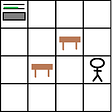## Mouse Cursor Control Using Facial Movements — An HCI Application## Using Computer Vision and Topic Modeling to Increase Brand Engagement on Instagram: The Case of…## Neural Networks Part 2: Implementing a Neural Network function in python using Keras## Deep CNN Architectures: ZFNet (ILSVRC Runner-up 2013)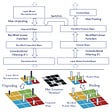## Depth-wise Separable Convolutional Neural Networks [CNN]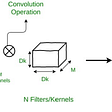## Paper review-AsynDGAN:Train deep learning Without Sharing Medical Image Data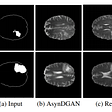## Evaluate your Recommendation Engine using NDCG## Hyugen AI

We work on statistics, machine learning, AI and we want to bring Artificial General Intelligence into the real world — Follow us https://twitter.com/HyugenAI

## Pruning in neural networks## Step by step guide to setup Tensorflow with GPU support on windows 10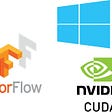## Deepfake Video Detection Using Long Short-Term Memory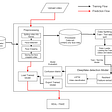## ASR(Speech Recognition) creation with Tranformers NN